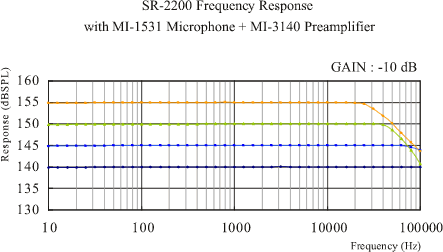Measurable frequency when the MI-1531 microphone is used with the SR-2200 microphone amplifier.Up to 100 kHz high frequency range can be measured when the MI-1531 microphone and the MI-3140 exclusive pre-amplifier are used with the SR-2200 2-ch amplifier. In addition, please note that measurable frequency range limit will be changed by the size of sound pressure to be measured.Explanation of the above frequency response figure   The figure shows the frequency response (representative value) when the GAIN setting of the SR-2200 2-ch amplifier is -10. From the relationship between the input sound pressure and the GAIN setting of the SR-2200, the frequency response can be estimate as the following examples of 1, 2 and 3. Example 1: When the GAIN setting is +10 and input sound pressure of the SR-2200 is 130 dB, the frequency response is almost the same as the input sound pressure of 140 dB in the figure. Example 2: When the GAIN setting is +10 and sound pressure of the SR-2200 is 140 dB, the frequency response is almost the same as the input sound pressure of 150 dB in the figure. Example 3: When the GAIN setting of the SR-2200 is + 20 or more, the relation between the frequency response can be considered as same as the above examples. But please note that the frequency response in the high range is worsened when the GAIN is increased.

Revised:2007.03.19